Home » 40 Questions to test a data scientist on Deep Learning [Solution: SkillPower – Deep Learning, DataFest 2017]

# 40 Questions to test a data scientist on Deep Learning [Solution: SkillPower – Deep Learning, DataFest 2017]

## Introduction

Deep Learning has made many practical applications of machine learning possible. Deep Learning breaks down tasks in a way that makes all kinds of applications possible. This skilltest was conducted to test your knowledge of deep learning concepts.

A total of 853 people registered for this skill test. The test was designed to test the conceptual knowledge of deep learning. If you are one of those who missed out on this skill test, here are the questions and solutions. You missed on the real time test, but can read this article to find out how you could have answered correctly.

Here are the leaderboard ranking for all the participants.

## Overall Scores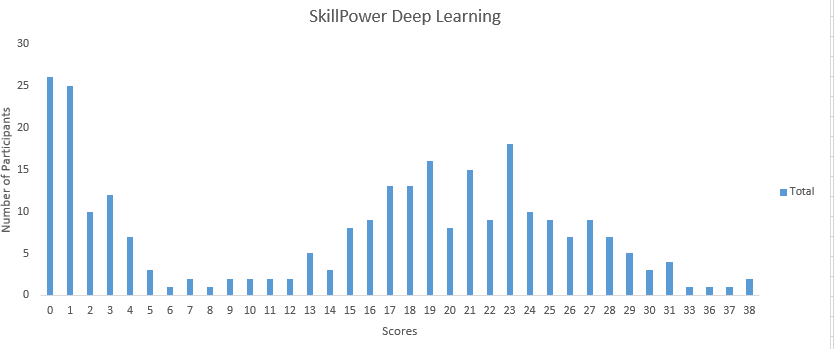You can access the final scores here. More than 270 people participated in the skill test and the highest score obtained was 38. Here are a few statistics about the distribution.

Mean Score: 15.05

Median Score: 18

Mode Score: 0

## Useful Resources

A Complete Guide on Getting Started with Deep Learning in Python

The Evolution and Core Concepts of Deep Learning & Neural Networks

Practical Guide to implementing Neural Networks in Python (using Theano)

Fundamentals of Deep Learning – Starting with Artificial Neural Network

An Introduction to Implementing Neural Networks using TensorFlow

Fine-tuning a Keras model using Theano trained Neural Network & Introduction to Transfer Learning

6 Deep Learning Applications a beginner can build in minutes (using Python)

1) The difference between deep learning and machine learning algorithms is that there is no need of feature engineering in machine learning algorithms, whereas, it is recommended to do feature engineering first and then apply deep learning.

A) TRUE

B) FALSE

2) Which of the following is a representation learning algorithm?

A) Neural network

B) Random Forest

C) k-Nearest neighbor

D) None of the above

3) Which of the following option is correct for the below-mentioned techniques?

2. L-BFGS uses second order differentiation
4. L-BFGS uses first order differentiation

A) 1 and 2

B) 3 and 4

C) 1 and 4

D) 2 and 3

4) Increase in size of a convolutional kernel would necessarily increase the performance of a convolutional neural network.

A) TRUE

B) FALSE

Question Context

Suppose we have a deep neural network model which was trained on a vehicle detection problem. The dataset consisted of images on cars and trucks and the aim was to detect name of the vehicle (the number of classes of vehicles are 10).
Now you want to use this model on different dataset which has images of only Ford Mustangs (aka car) and the task is to locate the car in an image.

5) Which of the following categories would be suitable for this type of problem?

A) Fine tune only the last couple of layers and change the last layer (classification layer) to regression layer

B) Freeze all the layers except the last, re-train the last layer

C) Re-train the model for the new dataset

D) None of these

6) Suppose you have 5 convolutional kernel of size 7 x 7 with zero padding and stride 1 in the first layer of a convolutional neural network. You pass an input of dimension 224 x 224 x 3 through this layer. What are the dimensions of the data which the next layer will receive?

A) 217 x 217 x 3

B) 217 x 217 x 8

C) 218 x 218 x 5

D) 220 x 220 x 7

Solution: (C)

7) Suppose we have a neural network with ReLU activation function. Let’s say, we replace ReLu activations by linear activations.

Would this new neural network be able to approximate an XNOR function?

Note: The neural network was able to approximate XNOR function with activation function ReLu.

A) Yes

B) No

Solution: (B)

If ReLU activation is replaced by linear activation, the neural network loses its power to approximate non-linear function.

8) Suppose we have a 5-layer neural network which takes 3 hours to train on a GPU with 4GB VRAM. At test time, it takes 2 seconds for single data point.

Now we change the architecture such that we add dropout after 2nd and 4th layer with rates 0.2 and 0.3 respectively.

What would be the testing time for this new architecture?

A) Less than 2 secs

B) Exactly 2 secs

C) Greater than 2 secs

D) Can’t Say

Solution: (B)

The changes is architecture when we add dropout only changes in the training, and not at test time.

9) Which of the following options can be used to reduce overfitting in deep learning models?

2. Use data augmentation
3. Use architecture that generalizes well
5. Reduce architectural complexity

A) 1, 2, 3

B) 1, 4, 5

C) 1, 3, 4, 5

D) All of these

Solution: (D)

All of the above techniques can be used to reduce overfitting.

10) Perplexity is a commonly used evaluation technique when applying deep learning for NLP tasks. Which of the following statement is correct?

A) Higher the perplexity the better

B) Lower the perplexity the better

Solution: (B)

11) Suppose an input to Max-Pooling layer is given above. The pooling size of neurons in the layer is (3, 3).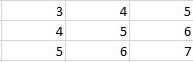What would be the output of this Pooling layer?

A) 3

B) 5

C) 5.5

D) 7

Solution: (D)

Max pooling works as follows, it first takes the input using the pooling size we defined, and gives out the highest activated input.

12) Suppose there is a neural network with the below configuration.

If we remove the ReLU layers, we can still use this neural network to model non-linear functions.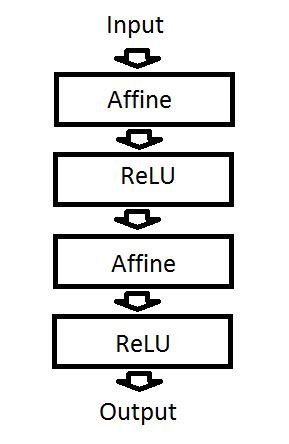A) TRUE

B) FALSE

Solution: (B)

13) Deep learning can be applied to which of the following NLP tasks?

A) Machine translation

B) Sentiment analysis

D) All of the above

Solution: (D)

Deep learning can be applied to all of the above-mentioned NLP tasks.

14) Scenario 1: You are given data of the map of Arcadia city, with aerial photographs of the city and its outskirts. The task is to segment the areas into industrial land, farmland and natural landmarks like river, mountains, etc.

Scenario 2: You are given data of the map of Arcadia city, with detailed roads and distances between landmarks. This is represented as a graph structure. The task is to find out the nearest distance between two landmarks.

Deep learning can be applied to Scenario 1 but not Scenario 2.

A) TRUE

B) FALSE

Solution: (B)

Scenario 1 is on Euclidean data and scenario 2 is on Graphical data. Deep learning can be applied to both types of data.

15) Which of the following is a data augmentation technique used in image recognition tasks?

1. Horizontal flipping
2. Random cropping
3. Random scaling
4. Color jittering
5. Random translation
6. Random shearing

A) 1, 2, 4

B) 2, 3, 4, 5, 6

C) 1, 3, 5, 6

D) All of these

Solution: (D)

16) Given an n-character word, we want to predict which character would be the n+1th character in the sequence. For example, our input is “predictio” (which is a 9 character word) and we have to predict what would be the 10th character.

Which neural network architecture would be suitable to complete this task?

A) Fully-Connected Neural Network

B) Convolutional Neural Network

C) Recurrent Neural Network

D) Restricted Boltzmann Machine

Solution: (C)

Recurrent neural network works best for sequential data. Therefore, it would be best for the task.

17) What is generally the sequence followed when building a neural network architecture for semantic segmentation for image?

A) Convolutional network on input and deconvolutional network on output

B) Deconvolutional network on input and convolutional network on output

Solution: (A)

18) Sigmoid was the most commonly used activation function in neural network, until an issue was identified. The issue is that when the gradients are too large in positive or negative direction, the resulting gradients coming out of the activation function get squashed. This is called saturation of the neuron.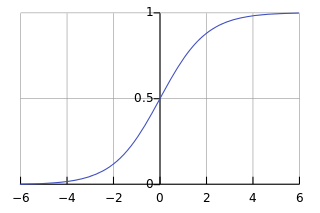That is why ReLU function was proposed, which kept the gradients same as before in the positive direction.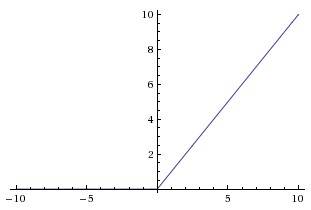A ReLU unit in neural network never gets saturated.

A) TRUE

B) FALSE

Solution: (B)

ReLU can get saturated too. This can be on the negative side of x-axis.

19) What is the relationship between dropout rate and regularization?

Note: we have defined dropout rate as the probability of keeping a neuron active?

A) Higher the dropout rate, higher is the regularization

B) Higher the dropout rate, lower is the regularization

Solution: (B)

Higher dropout rate says that more neurons are active. So there would be less regularization.

20) What is the technical difference between vanilla backpropagation algorithm and backpropagation through time (BPTT) algorithm?

A) Unlike backprop, in BPTT we sum up gradients for corresponding weight for each time step

B) Unlike backprop, in BPTT we subtract gradients for corresponding weight for each time step

Solution: (A)

BPTT is used in context of recurrent neural networks. It works by summing up gradients for each time step

21) Exploding gradient problem is an issue in training deep networks where the gradient getS so large that the loss goes to an infinitely high value and then explodes.

What is the probable approach when dealing with “Exploding Gradient” problem in RNNs?

A) Use modified architectures like LSTM and GRUs

C) Dropout

D) None of these

Solution: (B)

To deal with exploding gradient problem, it’s best to threshold the gradient values at a specific point. This is called gradient clipping.

22) There are many types of gradient descent algorithms. Two of the most notable ones are l-BFGS and SGD. l-BFGS is a second order gradient descent technique whereas SGD is a first order gradient descent technique.

In which of the following scenarios would you prefer l-BFGS over SGD?

1. Data is sparse
2. Number of parameters of neural network are small

A) Both 1 and 2

B) Only 1

C) Only 2

D) None of these

Solution: (A)

l-BFGS works best for both of the scenarios.

23) Which of the following is not a direct prediction technique for NLP tasks?

A) Recurrent Neural Network

B) Skip-gram model

C) PCA

D) Convolutional neural network

Solution: (C)

24) Which of the following would be the best for a non-continuous objective during optimization in deep neural net?

A) L-BFGS

B) SGD

Solution: (D)

Other optimization algorithms might fail on non-continuous objectives, but sub-gradient method would not.

25) Which of the following is correct?

1. Dropout randomly masks the input weights to a neuron
2. Dropconnect randomly masks both input and output weights to a neuron

A) 1 is True and 2 is False

B) 1 is False and 2 is True

C) Both 1 and 2 are True

D) Both 1 and 2 are False

Solution: (D)

In dropout, neurons are dropped; whereas in dropconnect; connections are dropped. So both input and output weights will be rendered in useless, i.e. both will be dropped for a neuron. Whereas in dropconnect, only one of them should be dropped

26) While training a neural network for image recognition task, we plot the graph of training error and validation error for debugging.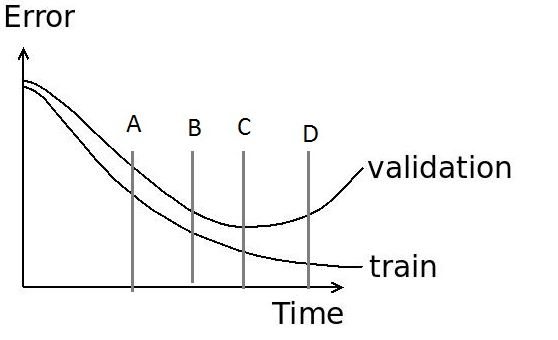What is the best place in the graph for early stopping?

A) A

B) B

C) C

D) D

Solution: (C)

You would “early stop” where the model is most generalized. Therefore option C is correct.

27) Research is going on to solve image inpainting problem using deep learning. For this, which loss function would be appropriate for computing the pixel-wise region to be inpainted?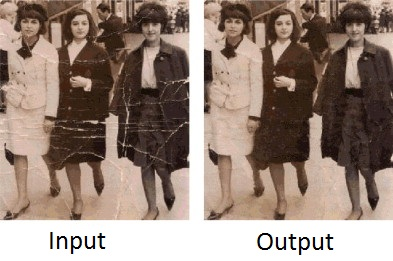Image inpainting is one of those problems which requires human expertise for solving it. It is particularly useful to repair damaged photos or videos. Below is an example of input and output of an image inpainting example.

A) Euclidean loss

B) Negative-log Likelihood loss

C) Any of the above

Solution: (C)

Both A and B can be used as a loss function for image inpainting problem.

28) Backpropagation works by first calculating the gradient of ___ and then propagating it backwards.

A) Sum of squared error with respect to inputs

B) Sum of squared error with respect to weights

C) Sum of squared error with respect to outputs

D) None of the above

Solution: (C)

29) Mini-Batch sizes when defining a neural network are preferred to be multiple of 2’s such as 256 or 512. What is the reason behind it?

A) Gradient descent optimizes best when you use an even number

B) Parallelization of neural network is best when the memory is used optimally

C) Losses are erratic when you don’t use an even number

D) None of these

Solution: (B)

30) Xavier initialization is most commonly used to initialize the weights of a neural network. Below is given the formula for initialization.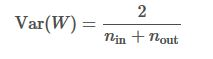1. If weights at the start are small, then signals reaching the end will be too tiny.
2. If weights at the start are too large, signals reaching the end will be too large.
3. Weights from Xavier’s init are drawn from the Gaussian distribution.

Xavier’s init helps reduce vanishing gradient problem.

Xavier’s init is used to help the input signals reach deep into the network. Which of the following statements are true?

A) 1, 2, 4

B) 2, 3, 4

C) 1, 3, 4

D) 1, 2, 3

E) 1, 2, 3, 4

Solution: (D)

All of the above statements are true.

31) As the length of sentence increases, it becomes harder for a neural translation machine to perform as sentence meaning is represented by a fixed dimensional vector. To solve this, which of the following could we do?

A) Use recursive units instead of recurrent

B)Use attention mechanism

C) Use character level translation

D) None of these

Solution: (B)

32) A recurrent neural network can be unfolded into a full-connected neural network with infinite length.

A) TRUE

B) FALSE

Solution: (A)

Recurrent neuron can be thought of as a neuron sequence of infinite length of time steps.

33) Which of the following is a bottleneck for deep learning algorithm?

A) Data related to the problem

B) CPU to GPU communication

C) GPU memory

D) All of the above

Solution: (D)

Along with having the knowledge of how to apply deep learning algorithms, you should also know the implementation details. Therefore you should know that all the above mentioned problems are a bottleneck for deep learning algorithm.

34) Dropout is a regularization technique used especially in the context of deep learning. It works as following, in one iteration we first randomly choose neurons in the layers and masks them. Then this network is trained and optimized in the same iteration. In the next iteration, another set of randomly chosen neurons are selected and masked and the training continues.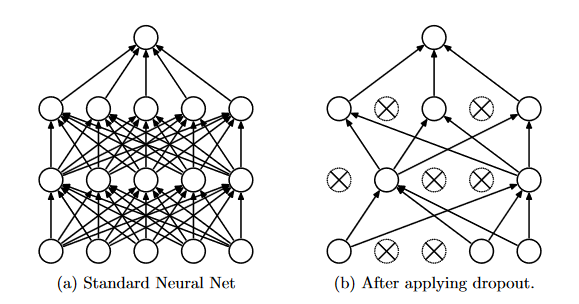Dropout technique is not an advantageous technique for which of the following layers?

A) Affine layer

B) Convolutional layer

C) RNN layer

D) None of these

Solution: (C)

Dropout does not work well with recurrent layer. You would have to modify dropout technique a bit to get good results.

35) Suppose your task is to predict the next few notes of song when you are given the preceding segment of the song.

For example:

The input given to you is an image depicting the music symbols as given below,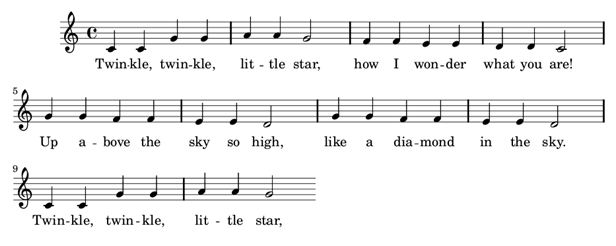Your required output is an image of succeeding symbols.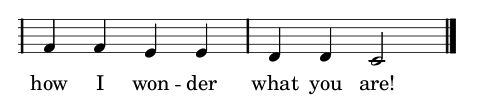Which architecture of neural network would be better suited to solve the problem?

A) End-to-End fully connected neural network

B) Convolutional neural network followed by recurrent units

C) Neural Turing Machine

D) None of these

Solution: (B)

CNN work best on image recognition problems, whereas RNN works best on sequence prediction. Here you would have to use best of both worlds!

36) When deriving a memory cell in memory networks, we choose to read values as vector values instead of scalars. Which type of addressing would this entail?

Solution: (A)

37) It is generally recommended to replace pooling layers in generator part of convolutional generative adversarial nets with ________ ?

A) Affine layer

B) Strided convolutional layer

C) Fractional strided convolutional layer

D) ReLU layer

Solution: (C)

Option C is correct. Go through this link.

Question Context 38-40

GRU is a special type of Recurrent Neural Networks proposed to overcome the difficulties of classical RNNs. This is the paper in which they were proposed: “On the Properties of Neural Machine Translation: Encoder–Decoder Approaches. Read the full paper here

38) Which of the following statements is true with respect to GRU?

1. Units with short-term dependencies have reset gate very active.
2. Units with long-term dependencies have update gate very active

A) Only 1

B) Only 2

C) None of them

D) Both 1 and 2

Solution: (D)

39) If calculation of reset gate in GRU unit is close to 0, which of the following would occur?

A) Previous hidden state would be ignored

B) Previous hidden state would be not be ignored

Solution: (A)

40) If calculation of update gate in GRU unit is close to 1, which of the following would occur?

A) Forgets the information for future time steps

B) Copies the information through many time steps

Solution: (B)

### End Notes

If you missed out on this competition, make sure you complete in the ones coming up shortly. We are giving cash prizes worth \$10,000+ during the month of April 2017.

If you have any questions or doubts feel free to post them below.

### Check out all the upcoming skilltests here.

You can also read this article on our Mobile APP•Asha Vishwanathan says:

Hi,
Thanks for the solutions. However, some doubts..
In Q.8, since dropout has no change in the test time, shouldn’t the answer be exactly 2 secs. ie. option B
Q.25, the explanation given maps to Q.24
Q.33, while the explanation says “Therefore you should know that all the above mentioned problems are a bottleneck for deep learning algorithm”, the answer chosen in option A.

•Faizan Shaikh says:

Thanks Asha for the feedback; updated them

•May says:

As a newbie to deep learning, I wonder to crack these questions, what material is the best in terms of the understanding the application of and the tuning of deep learning projects. Thanks.

•Faizan Shaikh says:

Explanations and resources are regularly published at Analytics Vidhya on Deep Learning and Data Science in general. You can refer them if you want.